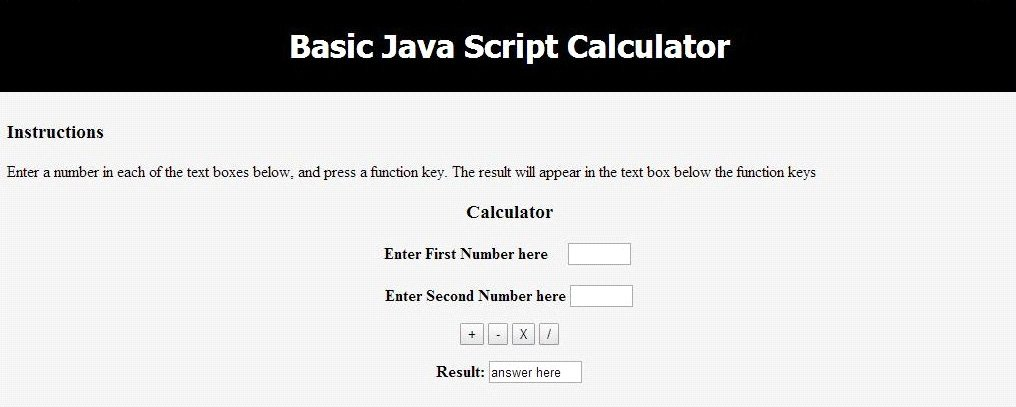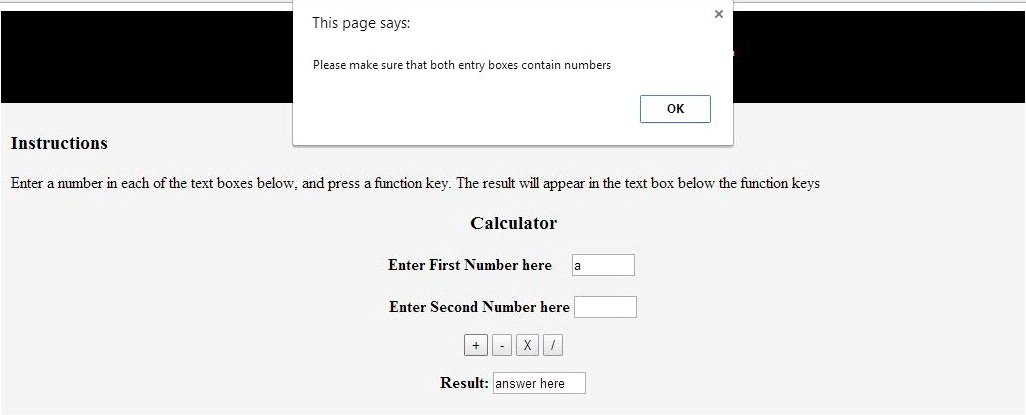# Javascript Code Examples: FOUR FUNCTION CALCULATOR

Introduction: The aim of this project is to create a four function calculator using JavaScript programming language. The calculator designed performs addition, subtraction, multiplication and division of tow given numbers.

Code Implementation:

After the basic HTML declarations, we define the type attribute which specifies the type of document for the Internet media. In this project we define `<style type="text/css">` which means that the content is CSS.

There is a function “ErrorCheck” defined in the next step. This function will check if the input numbers are proper numerals. ErrorCheck is called in the calculator functions. ErrorCheck works as follows: if there is no number entered in the input field or if the value entered in the input field is not a number then the function returns a Boolean FALSE which displays a dialog box to the user stating “Please make sure that both entry boxes contain numbers”. If the input value entered is a number in both the fields then the Boolean TRUE is returned.

Next piece of code implemented is the the form “theCalculator”. This consists of all the functions that are called for computation : AddNumbers, SubtractNumbers, MultiplyNumbers and DivideNumbers

All the four functions described first check for the input value and then convert the value inputed as number. This is done because we get the input as text.

SubtractNumbers function: subtracts two given number
MultiplyNumbers function: multiplies two given number
DivideNumbers function: divides the two given number

Next the HTML form is written with the script embedded. It takes in the input numbers and calls the functions described above accordingly.

Test Output:

After the execution of the file the first page displayed is:Error Screen: when the input values are null or non-numerical## Help with JavaScript Assignments

You’ve read one of our Javascript code examples. If you need help with JavaScript or any other any type of IT sample assignments, you can contact us any time needed. We specialize in various technical disciplines and can complete project of any difficulty level for you.

By getting Java Script online help from us, you get solution ideas, explanations and just professional assistance from experienced experts.